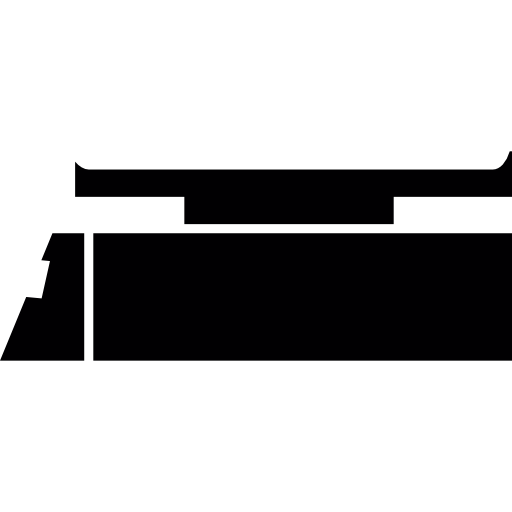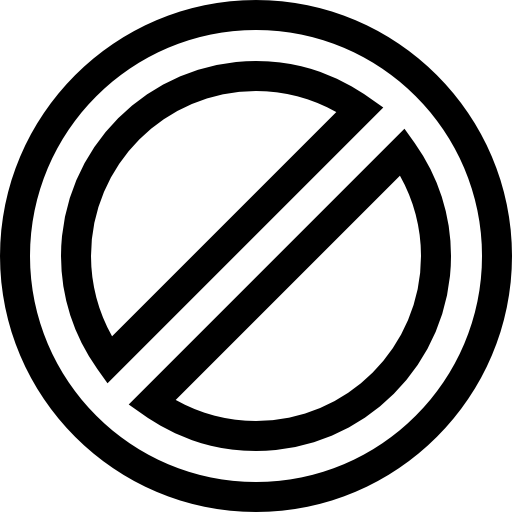CNC Illustration by Gwendolyn Say (@saydrawings)

# Stoichiometry

Stoichiometry empowers us with the mathematical tools to know precisely the number of particles present in a sample before us. It also allows us to gaze into the future as we predict the amount of products without a crystal ball.

# SUMMARY### Mole

1 mole is 6×1023 particles. Stoichiometry means the mole ratio of substances in a reaction.

no of moles = no of particles ÷ 6×1023### Relative Molecular Mass

The mass of a molecule compared to 1/12th of a carbon-12 atom.

Mr of AnBm = (n × Ar of A) + (m × Ar of B)### Molecule

The mass of 1 mol of a substance, numerically equal to the Ar or Mr.

molar mass = mass in g ÷ no of moles### Percentage Mass

The percentage by mass of a compound that is made up of an element.

% mass of A in AnBm= nAr ÷ Mr × 100%### Empirical Formula

The simplest ratio of atoms of each element in a compound.

1. Write the masses of each element
2. Divide by Ar
3. Divide by smallest number### Molecular Formula

Shows the number of atoms of each element in one simple molecule.

1. Find n = Mr ÷ mass of empirical formula
2. Multiply numbers in empirical formula by n### Molar Volume

A mole of gas occupies 24 dm3 of volume at room temperature and pressure (rtp).

no of moles = gas volume in dm3 ÷ 24### Solution Concentration

The number of moles or mass of solutes per dm3 of solvent.

concentration = no of moles ÷ volume in dm3
concentration = mass ÷ volume in dm3### Titration

Carefully adding exact amount of one solution to completely react with another.

1. Find no of moles of titrant = concentration × volume
2. Use mole ratio to find no of moles of unknown
3. Find conc of unknown = no of mol ÷ volume### Limiting Reactant

The reactant that is completely used up, limiting the amount of product.

1. Find the no of moles of reactants A and B provided
2. Find the no of moles of B needed if A were used up
3. A is limiting if there is less B needed than provided### Percentage Yield

The extent and efficiency of converting reactants to products.

% yield = expected yield ÷ theoretical yield × 100%### Percentage Purity

The percentage by mass of an impure mixture that comprises the pure substance.

% purity = mass of pure substance ÷ mass of impure mixture × 100%

## Percentage mass & empirical formula

Calculate how each element in a compound is pulling its weight, and use this to find the chemical formula empirically

## Stoichiometry: Mole ratio & limiting reactant

Look into the future and predict the amount of product formed, by identifying the mole ratio and limiting reactant

## Stoichiometry & the mole concept TYS questions

Fresh from the furnace of O Level

## Stoichiometry TYS questions – Combined Chemistry

There is no stoichiometry without try: try out questions from the O Level Combined Chemistry papers

## Solution concentration & titration

Sorry, we don't have 25% sugar here. How about 100 g/dm3 or 0.292 mol/dm3?

## Relative atomic mass & molecular mass

Unit-less but meaningful: making sense of the mass of an atom with ratio

## The mole & molar mass

Learn how how to count, but in moles

## Salt preparation TYS questions

It's big brain time, as you think backwards from the salt in question to infer the reagents and method

## Molar volume of gases

Gases of different masses taking up the same spaces

## Practise how to write chemical formula

Can you infer the formulae of ionic compounds, based on the charges of their constituent ions?

## Stoichiometry & the mole concept prelim questions

Learn stoichiometry and the mole concept by solving problems taken from O Level prelim papers. Answers and explanations are included.

## Stoichiometry: Percentage yield & percentage purity

Be 100% sure about how to calculate percentage yield and percentage purity